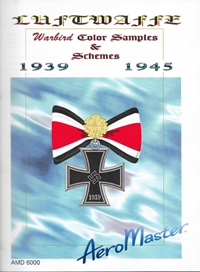## Reference Details for Luftwaffe Warbird Color Samples & Schemes 1939-1945Title Luftwaffe Warbird Color Samples & Schemes 1939-1945 Authors Publisher AeroMaster Year 2000 Types Covered Ar 234B, Bf 109E, Bf 109F, Bf 109G, Bf 109K, Bf 110C, Bf 110D, Bf 110E, Bf 110G, Do 17Z, Fw 190A, Fw 190D, Fw 190F, He 51B, He 111H, He 112, He 162A, Hs 129B, Ju 87B, Ju 87D, Ju 87R, Ju 88A, Ju 88G, Ju 188A, Ju 188F, Me 163B, Me 262A, Me 262B, Me 410 Cockpit Details Wheel Well Details Other Details Cutaway Drawings Multiview Drawings Generic Camouflage Patterns Ar 234B (p. 26), Bf 109E (p. 23), Bf 109F (p. 23, 24), Bf 109G (p. 24), Bf 109K (p. 24), Bf 110C (p. 26), Bf 110D (p. 26), Bf 110E (p. 26), Bf 110G (p. 25, 26), Do 17Z (p. 27), Fw 190A (p. 24), Fw 190D (p. 24), Fw 190F (p. 24), He 111 (p. 27), He 162A (p. 25), Ju 87B (p. 27), Ju 87D (p. 27), Ju 87R (p. 27), Ju 88A (p. 26), Ju 188 (p. 27), Me 163B (p. 25), Me 262A (p. 25), Me 262B (p. 25), Me 410 (p. 26) Generic Markings Patterns Ar 234B (p. 26), Bf 109E (p. 23), Bf 109F (p. 23, 24), Bf 109G (p. 24), Bf 109K (p. 24), Bf 110C (p. 26), Bf 110D (p. 26), Bf 110E (p. 26), Bf 110G (p. 25, 26), Do 17Z (p. 27), Fw 190A (p. 24), Fw 190D (p. 24), Fw 190F (p. 24), He 111 (p. 27), He 162A (p. 25), Ju 87B (p. 27), Ju 87D (p. 27), Ju 87R (p. 27), Ju 88A (p. 26), Ju 188 (p. 27), Me 163B (p. 25), Me 262A (p. 25), Me 262B (p. 25), Me 410 (p. 26) Specific Markings Profiles Ar 234B-1 (p. 17), Ar 234B-2 (p. 17), Bf 109E-1 (p. 1, 3), Bf 109E-3 (p. 1-3), Bf 109E-4 (p. 1-3), Bf 109F-2 (p. 4, 6, 7), Bf 109F-4 (p. 4, 6), Bf 109G-2 (p. 4-6), Bf 109G-6 (p. 4-7), Bf 109G-6/AS (p. 5), Bf 109G-10 (p. 5), Bf 109G-14 (p. 6), Bf 109K-4 (p. 46), Bf 110C (p. 13), Bf 110D-3 (p. 13, 14), Bf 110E-1 (p. 15), Bf 110G-2 (p. 14), Bf 110G-4 (p. 13, 14), Do 17Z (p. 19), Fw 190A-3 (p. 9, 10), Fw 190A-4 (p. 9, 10), Fw 190A-6 (p. 8), Fw 190A-7 (p. 8), Fw 190A-8 (p. 8-10), Fw 190D-9 (p. 11), Fw 190D-13 (p. 11), Fw 190F-8 (p. 10), He 51B (p. 18), He 111H-6 (p. 20), He 111H-14 (p. 20), He 111H-22 (p. 20), He 112 (p. 12), He 162A (p. 12), Hs 129B-1 (p. 17), Ju 87B-2 (p. 22), Ju 87D-3 (p. 22), Ju 87R-2 (p. 22), Ju 88A-1 (p. 18), Ju 88A-4 (p. 21), Ju 88G-6 (p. 21), Ju 188A-2 (p. 21), Ju 188F-2 (p. 21), Me 163B (p. 12), Me 262A-1a (p. 16), Me 262A-2a (p. 16), Me 262B-1 (p. 16), Me 262B-1a/U1 (p. 16), Me 410A (p. 15), Me 410B (p. 15) Notes